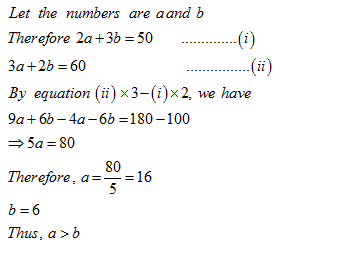• This is an assessment test.
• These tests focus on the basics of Maths and are meant to indicate your preparation level for the subject.
• Kindly take the tests in this series with a pre-defined schedule.

## Basic Maths: Test 10

Congratulations - you have completed Basic Maths: Test 10. You scored %%SCORE%% out of %%TOTAL%%. You correct answer percentage: %%PERCENTAGE%% . Your performance has been rated as %%RATING%%
 Question 1
The bus fare for one person from Agra to Aligarh is Rs.210 and the train fare between the same places for one person is equal to three-fourth the bus fare for two persons between the same places. What is the total fare paid by 2 persons travelling by bus and 4 persons travelling by train between the two places?
 A Rs.1680 B Rs.1560 C Rs.1440 D Rs.1306
Question 1 Explanation:
$\displaystyle \begin{array}{l}Bus\,\,fare\,=Rs.\,\,210\\Train\,\,fare\,=2\times 210\times \frac{3}{4}\\=Rs.\,315\\Therefore\,\,\operatorname{Re}quired\,\,\,total\,\,fare\,\\=Rs.\,\left( 2\times 210+4\times 315 \right)\\=Rs.\,\left( 420+1260 \right)\\=Rs.\,1680\end{array}$
 Question 2
There are two numbers such that the sum of twice the first number and thrice the second number is 50 and the sum of thrice the first number and twice the second number is 60.Which is the larger number?
 A 32 B 12 C 14 D 16
Question 2 Explanation:Question 3
$\displaystyle 18.5\times 21.4\times p=6255.22$
what is the value of P
 A 15.8 B 14.6 C 17.4 D 17.2
Question 3 Explanation:
$\displaystyle p=\frac{6255.22}{18.5\times 21.4}=15.8$
 Question 4
$\displaystyle 1.5\times 78\div 0.5=a$
what is the value of P
 A 238 B 234 C 243 D 216
Question 4 Explanation:
$\displaystyle a=\frac{1.5\times 78}{0.5}=234$
 Question 5
120 = p% of 800
what is the value of P
 A 6 B 12 C 20 D 15
Question 5 Explanation:
120 = p% of 800
p = 120/8 = 15
Once you are finished, click the button below. Any items you have not completed will be marked incorrect.
There are 5 questions to complete.
 ← List →